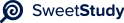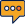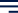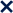•01 / 5100%1
APPLYING PROBABILITY DISTRIBUTIONS
Lisa Ayers
Walden University
Professor: Kaplan
Stat 2002
10/29/20232
APPLYING PROBABILITY DISTRIBUTIONS
1. A market-research firm was hired to determine the percentage of people in a market area
who would purchase a client's magazine if a door-to-door sales campaign were
undertaken. The firm stated that 40% would buy if contacted at home. Suppose the
marketing company has tried the sales campaign at 300 randomly selected homes.
Assuming the market research was done properly and the 40% is representative, how
many sales are expected if the publisher attempts to sell to 5,000 homes?
If the market research was done properly, and 40% represents the true probability of
purchase, we can use the binomial distribution formula to find the expected number of
sales if the publisher attempts to sell to 5,000 homes. Expected Sales = (Probability of
purchase) x (Number of homes) Expected Sales = (0.40) x (5,000) = 2,000 sales. So, if
the campaign is conducted with 5,000 homes, the expected number of sales is 2,000.
If the market research was accurate, what is the probability that fewer than 100
individuals will buy? Use the normal approximation to the binomial.
To find the probability that fewer than 100 individuals will buy, we can use the normal
approximation to the binomial distribution because the number of trials (5,000) is large, and the
probability of
success (0.40) is not too small or too large First, calculate the mean (μ) and standard
deviation (σ) of the binomial distribution:@
μ = n * p@
@ @= 5,000 * 0.40@
@ @ = 2,000@
σ = √(n * p * (1 - p))@ @
@= √(5,000 * 0.40 * 0.60)@
@≈ 40
Now, calculate the z-score for 100 individuals:@
z = (X - μ) / σ@
z = (100 - 2,000) / 40@
@ ≈ -47.53
Using a standard normal distribution table or calculator, the probability P(Z < -47.5) is
extremely close to 0. So, the probability of fewer than 100 individuals buying is nearly zero.
Suppose the publisher actually sells the magazine to 70 people out of the 300
This suggests that the market research estimate of 40% was likely overstated.@
Additionally, the campaign's performance fell significantly short of the expected 120 sales (40%
of 300).@
This may indicate that the market research was not an accurate representation of the target
market and the campaign strategy may need to be reevaluated.
2. A steel mill produces alloy sheets used for the bodies of automobiles. The mill produces
sheets with an average thickness of 0.517 inches and a standard deviation of 0.037
inches. A new car model requires alloy sheets between 0.495 and 0.525 inches thick.
What percentage of the sheets made by the mill will be suitable for the new car model?
To find the percentage of alloy sheets suitable for the new car model (0.495 to 0.525
inches thick),@we can calculate the z-scores for both thickness limits and find the area
under the normal@distribution curve between those z-scores. μ (mean thickness) = 0.517
inches@σ (standard deviation) = 0.037 inches
@
For X = 0.495 inches:@
z = (0.495 - 0.517) / 0.037@
@ @≈ -0.5959
@
For X = 0.525 inches:@
z = (0.525 - 0.517) / 0.037@
@ ≈ 0.2162
@
Using a standard normal distribution table or calculator, we can find the area between
these two z-scores. P(-0.5959 < Z < 0.2162) ≈ 0.34094
@ So, approximately 34.09% of the sheets produced by the mill will be suitable for the new
car model.
3. The VP of HR for a large company is interested in the distribution of sick-leave hours for
employees at the company. A recent study revealed that the distribution was consistent
with a normal model, with a mean of 58 hours per year, and a standard deviation of 14
hours. An office manager of one division believes that during the past year, two of the
division's employees have taken excessive sick leave. One took 74 hours and the other
used 90 hours. What would you conclude about the division manager's claim, and why?
To evaluate the division manager's claim about excessive sick leave, we can calculate the
z-scores for the@two employees who took 74 and 90 hours of sick leave.
@@ Mean (μ) = 58 hours@
Standard deviation (σ) = 14 hours
@
For the employee who took 74 hours:@
z = (74 - 58) / 14 ≈ 1.143
@ For the employee who took 90 hours:@
z = (90 - 58) / 14 ≈ 2.286
@
Now, using a standard normal distribution table or calculator, we can find the
probabilities associated with these z-scores.
@
* For a z-score of 1.143, P(Z < 1.143) ≈ 0.8708.
* For a z-score of 2.286, P(Z < 2.286) ≈ 0.9885.
@
Both probabilities are relatively high, suggesting that these sick leave hours are not
extremely unusual@based on the normal distribution.@Therefore, the division manager's
claim does not have strong statistical support, and the sick leave taken@by these
employees may not be considered excessive within the context of the distribution.
@
4. Each month, an American household generates an average of 28 pounds of newspaper for
garbage and/or recycling. Assume this is approximately normally distributed and that the
standard deviation is 2 pounds. If a household is selected at random, find the probability
of it generating between 27-31 pounds per month?5
For the normally distributed variable of newspaper weight with a mean (μ) of 28 pounds
and a standard@deviation (σ) of 2 pounds, we want to find the probability of a household
generating between 27@
and 31 pounds per month.
@
(a) Calculate the z-scores for the lower and upper limits:@
@
For X = 27 pounds:@
z = (27 - 28) / 2 = -0.5
@
For X = 31 pounds:@
z = (31 - 28) / 2 = 1.5
@
(b) Now, find the area under the normal distribution curve between these z-scores:
P(-0.5 < Z < 1.5) ≈ P(Z < 1.5) - P(Z < -0.5)
@
Using a standard normal distribution table or calculator, P(Z < 1.5) ≈ 0.9332, and P(Z < -
0.5) ≈ 0.3085.
P(-0.5 < Z < 1.5) ≈ 0.9332 - 0.3085 ≈ 0.6247
@
So, the probability of a household generating between 27 and 31 pounds of newspaper@
per month is approximately 62.47%.
@